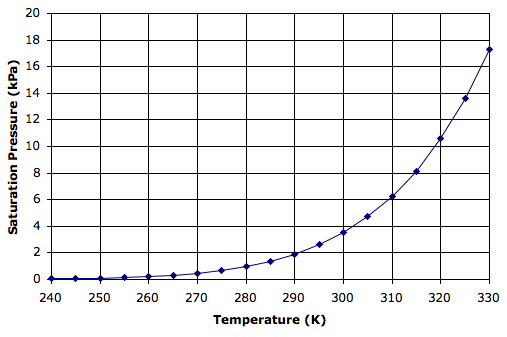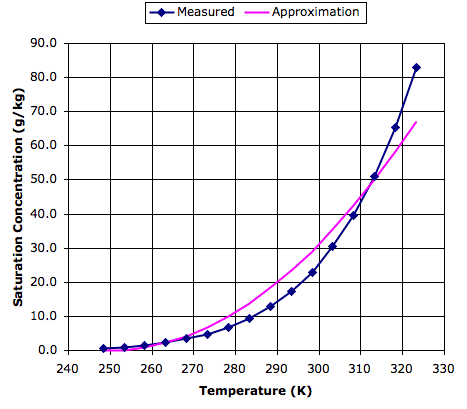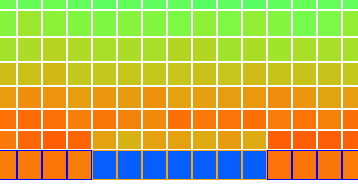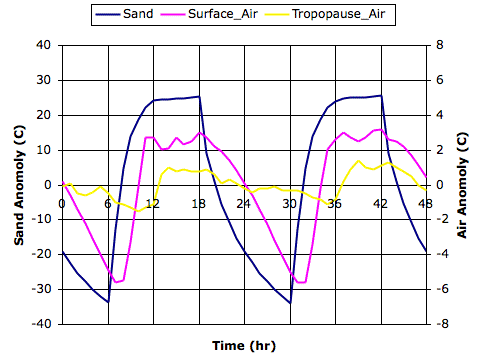## Thursday, August 25, 2011

### Condensation Point

Suppose a body of moist air rises from the surface of the sea. The weight of air pressing down upon it decreases as it rises. Its pressure drops. It expands and cools adiabatically (see Adiabatic Balloons). So long as no condensation occurs, its temperature drops by 1°C for each 100 m that it ascends (see Tempearture, Pressure, and Altitude). Will the water vapor eventually condense into droplets?

If we know the initial concentration of water vapor in grams of water per kilogram of air, this concentration will remain constant as the air moves upwards. As the temperature of the air drops, however, perhaps there will come a time when the concentration of water vapor exceeds the saturation concentration, and at that point droplets will form. In Evaporation Rate we presented a graph of saturation concentration versus air temperature. But the data points of this graph correspond to measurements taken on the surface of the Earth, where air pressure is 100 kPa. What will the saturation concentration be at the lower pressures that apply to our rising body of air?

The pressure exerted by a gas is the force exerted by its molecules bouncing off whatever surface they encounter. The pressure of moist air is the sum of the pressures exerted by its nitrogen, oxygen, water, and all other molecules. The pressure exerted by the nitrogen molecules is the partial pressure of nitrogen. The pressure exerted by the water molecules is the partial pressure of water. It turns out that the maximum possible partial pressure of water molecules depends only upon temperature. This maximum is the saturation pressure of water at a particular temperature. Regardless of the other gases that might be mixed with the water vapor, the saturation pressure is always the same at a particular temperature. The graph below gives saturation pressure versus temperature, as indicated by an empirical formula we found here.Water vapor is a gas just like nitrogen and oxygen. Its pressure depends only upon its volume and temperature. Water vapor in moist air will be at the same temperature as the air. It will share the same volume as the air. If the air pressure halves, so does the partial pressure of water vapor. For concentrations below a few percent, the concentration of water vapor at various air pressures is given by:

x = pwRw/pR = 0.62 pw/p,

where x is the concentration of water vapor, pw is its partial pressure, Rw is its specific gas constant, or 462 J/kgK, p is the air pressure, and R is the specific gas constant for air, or 287 J/kgK.

Consider moist air near the surface of the Earth at temperature 300 K, pressure 100 kPa, and water vapor partial pressure 2 kPa, which corresponds to concentration 12 g/kg. The water vapor pressure is roughly half the saturation pressure of 3.8 kPa shown on our graph. Suppose our moist air rises to 2000 m. Its cools to 280 K (1 K per 100 m) and its pressure drops to 80 kPa (adiabatic expansion of air). The concentration of water vapor remains 12 g/kg and its partial pressure drops slightly to 1.6 kPa. At 280 K, however, the saturation pressure has dropped all the way to 1 kPa, which corresponds to a concentration of only 7.6 g/kg. Thus each kilogram of air contains 4.4 g more water than it can hold as water vapor. This excess water must condense to form water droplets.

We would like a simple formula that will allow us to calculate the amount of water that must condense from moist, rising air in our Circulating Cells program. We combine the saturation concentration approximation of our Evaporation Rate post with the specific gas constants of air and water vapor, and with the assumption that the water vapor concentration is small, to arrive at the following approximation.

xs = (T−250)2p/8000

Here xs is the saturation concentration of water vapor in g/kg, p is the air pressure in kPa, and T is the temperature of the air in K. At 280 K and 80 kPa, this formula gives us 9 g/kg, which corresponds to a partial pressure of 1.2 kPa. This 1.2 kPa is close enough to the 1.0 kPa shown in the graph above.

The partial pressure of water vapor in moist air decreases as the air rises. But at the same time, the rising air cools by adiabatic expansion, and this cooling depresses the saturation pressure of water vapor so rapidly that condensation will eventually take place. With our approximate formula for saturation concentration with pressure and temperature, we will be able to simulate the condensation of water in moist, rising air.

## Wednesday, August 17, 2011

### Evaporation Rate

We are preparing to add evaporation from surface water to our Circulating Cells program. Water will leave the surface and enter the atmosphere as water vapor. The rate at which water evaporates depends upon the humidity and movement of the air above. The following empirical equation, which we found here, tells us the approximate rate at which water will evaporate into air, assuming the air is at roughly the same temperature as the water.

w = (0.007 + 0.005v)(xsx),

where w is the evaporation rate in grams per second for each square meter of water surface (g/m2s), v is the velocity of the air in meter per second (m/s), xs is the saturation concentration of water vapor in grams of water per kilogram of dry air (g/kg) for air at the same temperature as the water, and x is the actual concentration of water vapor in grams per kilogram of dry air (g/kg) in the air above the water.

In our simulation, we know the temperature of the surface water, and when we simulate a planet with a water surface using CC8, we find that the surface gas cells are within a few degrees of the temperature of the surface water. Our previous work on impetus for circulation suggests that the velocity of our gas cells is of order 4 m/s. When we implement evaporation, we will keep track of the water vapor concentration in each cell, so we will know x. What remains for us to determine is xs, the saturation concentration of water vapor in air at the surface temperature.

The following graph shows measured values of saturation concentration in g/kg for a range of temperatures in Kelvin, based upon data we found here. To see the same plot in Centigrade, see here.Also plotted on the graph is a parabolic approximation to the measured data, which is based upon two reference points: 0 g/kg at 250 K and 45 g/kg at 310 K. This approximation is good enough for our purposes, and will simplify our program. Thus our evaporation equation becomes:

w = [(T−250)2/80 − x] / 40

For example, if we have dry air over a lake at 290 K (14°C), water will evaporate at 0.5 g/m2s. In one hour, 1.8 kg of water will evaporate from each square meter. Our gas cells have mass 330 kg/m2, so after an hour over the lake, the gas will acquire water vapor concentration 5 g/kg, which is well below the saturation concentration of 20 g/kg given by our approximation. Its relative humidity will be 25%.

## Wednesday, August 10, 2011

### Island Inversion

We invite you to download Circulating Cells, Version 8.4 by clicking here so that you can watch it simulate air movement above an island in the sea. Start the program and set it to Cycle mode with Q_sun set to 700 W/m2. Download this file and read it into the simulation with the Load button. You will see the an island of sand at the center of the surface blocks, surrounded by water on the left and right. The saved state of the cell corresponds to midnight, so you can start the simulation right away.

Just before dawn, at solar time 5.5 hr, you will see that the temperature of the air resting upon the sand of the island is slightly cooler than the air above. The following figure is a close-up of the air above the sand, in which ran the program with max_T set to 300 K and min_T set to 260 K in the source code so as to make the cool layer more obvious. Note that the orange-lined surface blocks are sand and the blue-lined ones are water.Our simulation produces the inversion of atmospheric temperature that we discussed in Surface Cooling, Part IV. In that post, we proposed that the leaves of a forest would cool at night by radiation. Air in contact with the leaves would descend to the ground and drag warmer air down from above. We suggested that this process might cool the first ten to a hundred meters of air above the treetops, producing temperature inversion. Perhaps that is indeed what happens over a forest. But in our simulation, whenever the surface is cooler than the air resting upon it, we set the heat transfer by convection and conduction to zero. How, then, does inversion occur over our simulated island?

The sandy island cools by roughly 50°C to −12°C at night. At this temperature, it radiates only 260 W/m2. Of this, 130 W/m2 is absorbed by the bottom layer of the atmosphere, because we have transparency fraction set to 0.5. Meanwhile, the bottom layer of our simulated atmosphere radiates heat both night and day. At the start of the night, its temperature is around 300 K and it radiates 230 W/m2 to the sand below, which is half the heat a black body at that same temperature would radiate. By radiation alone, we see that the cells above the sand are losing 100 W/m2.

The mass of our cells is 330 kg/m2, and their heat capacity at constant pressure is 1 kJ/kg, so 100 W/m2 will cool them by roughly 1°C/hr. This is what we see during the night above our island. Inversion does not occur over the water because the water surface in our simulation has ten times the heat capacity as the sand, and so cools ten times less at night. The water continues to warm the lower atmosphere with its radiation during the night. Thus we see how back-radiation and a surface with low heat capacity work together to produce temperature inversion.

## Wednesday, August 3, 2011

In our Rotating Greenhouse post, we use CC5 to simulate the alternation between day and night by varying the Solar power delivered to the gas cells resting upon our simulated planet surface. But we did not simulate the surface itself, nor did we distinguish between the temperature of the surface gas and the radiating temperature of the planet surface: we used the same temperature. But the CC8 program we introduced in our previous post does simulate the planet surface, so we can see how the temperature of the sand itself varies with day and night.

During the day, most of the heat passing into the surface gas cells does so by convection. But convection occurs only when the gas above is cooler than the surface below. In our discussion of atmospheric inversion we saw how the ground can be colder than the surface air at night, which can lead to a pocket of cold air sitting near the ground, with warmer air up above. In our simulation, we set the convection transfer to zero when the surface is colder than the surface gas.

At night, therefore, a sandy surface will radiate its heat into space, and receive no warmth from the sun. But it will receive warmth from the atmosphere, in the form of the back-radiation we described in our previous post. During the day, we found that our surface gas was radiating 226 W/m2 down to the surface. This radiation will slow the cooling of the surface at night.

We ran our simulation with Cycle heating on a sandy planet surface, 700 W/m2 solar heat during the day, daylight fraction set to 0.50, convection coefficient 20 W/m2, and transparency fraction 0.50. You will find the equilibrium state of the cell array at midnight stored in a text file here. The following graph shows the average temperature of the sand blocks, the surface gas cells, and the tropopause gas cells during two complete day-night cycles. We plot the deviation of each temperature from its average value during the cycles, which is why we call the plots "anomalies".The temperature of the surface sand varies by almost 50°C, dropping as low as −12°C just before dawn. The temperature of the air a hundred meters above the sand, at the center of the bottom row of gas cells, varies by 9°C, dropping as low as 23°C. The tropopause responds far less to the day-nigh cycle, with a variation of only 2°C. These results are consistent with our observations of the desert, which we discussed at length in our Surface Cooling posts.

When we turn off the back-radiation in our simulation, the temperature of the surface sand drops by another 50°C at night, in a manner reminiscent of the Moon. And so we conclude that our atmosphere, by means of back-radiation, keeps us warm at night.